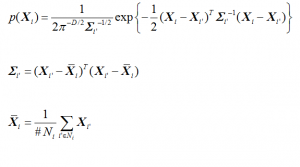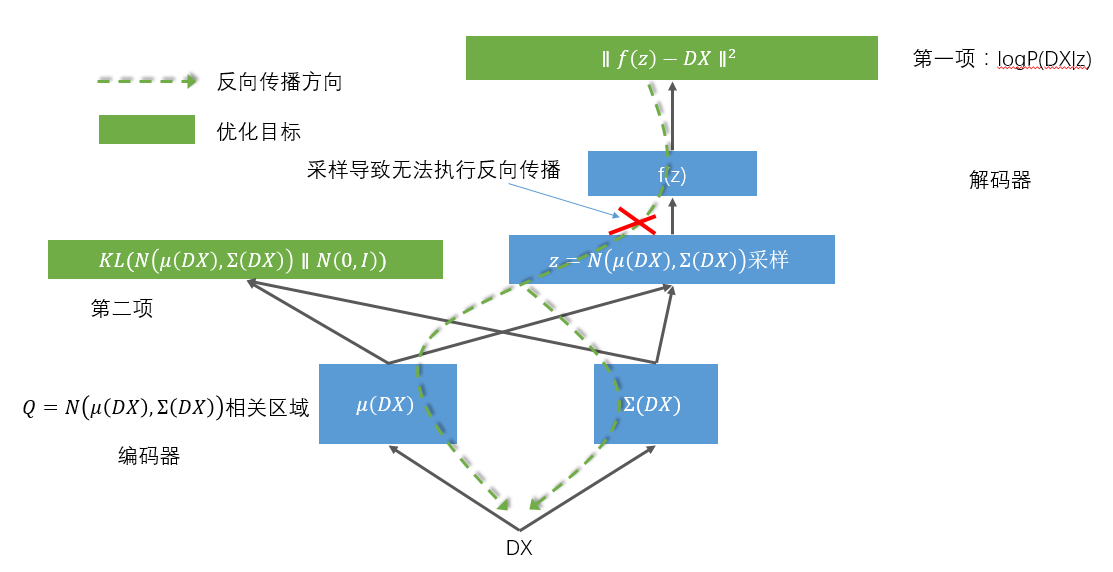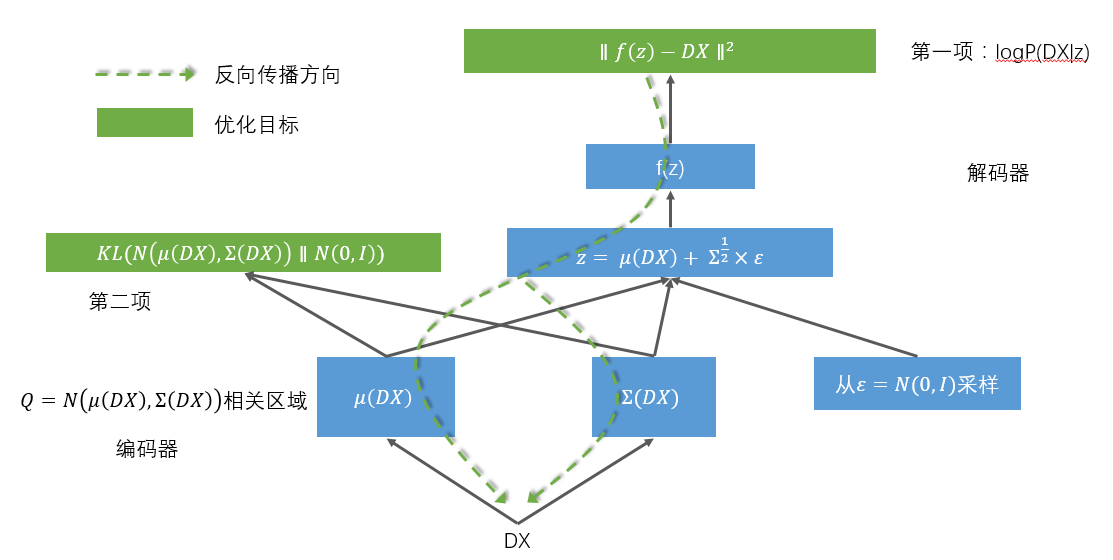# 变分自编码器（Variational Autoencoder, VAE）通俗教程

### 1. 神秘变量与数据集

$$z = \left( \begin{array}{c} z_1\\ z_2\\ \vdots\\ z_n \end{array} \right)$$

z也起个名字叫神秘组合

$$p(x|\mu,\sigma^2) = \frac{1}{\sqrt{2\pi}\sigma}e^{-\frac{1}{2}(\frac{x-\mu}{\sigma})^2}$$

$$p(x|\sigma^2) = \frac{1}{\sqrt{2\pi}\sigma}e^{-\frac{x^2}{2\sigma^2}}$$$$P_t(DX) = \int P_{xz}(DX|z;\theta)P_z(z)dz$$; (1)

OK，我们还要说一个关键问题，就是我们确信f是存在的，我们认为变量与神秘变量之间的关系一定可以用一个函数来表示。

### 2. 变分自编码器(VAE)

$$P_z(z) = N(0, I)$$,

$$P_{xz}(DX|z;\theta) = N(DX|f(x;\theta), \sigma^2 * I)$$,

$$P_t(DX) = \int P_{xz}(DX|z;\theta)P_z(z)dz$$,

### 3. 设定目标函数

$$KL(p \parallel q) = \sum p(x)log \frac{p(x)}{q(x)}$$

$$KL(p \parallel q) = \int p(x)log \frac{p(x)}{q(x)}dx$$

$$P_z(z|DX)$$和$$Q(z|DX)$$的KL散度为

$$\mathcal{D}[Q(z|DX)\parallel P_z(z|DX)] = \int Q(z|DX)[logQ(z|DX) – logP_z(z|DX)]$$

$$\mathcal{D}[Q(z|DX)\parallel P_z(z|DX)] = E_{z\sim Q}[logQ(z|DX) – logP_z(z|DX)]$$

$$P_z(z|DX) = \frac{P(DX|z)P(z)}{P(DX)}$$

$$\mathcal{D}[Q(z|DX)\parallel P_z(z|DX)] = E_{z\sim Q}[logQ(z|DX) – logP(DX|z) – logP(z)] + logP(DX)$$

$$logP(DX) – \mathcal{D}[Q(z|DX) \parallel P(z|DX)] = E_{z\sim Q}[logP(DX|z)] – \mathcal{D}[Q(z|DX) \parallel P(z)]$$; (2)

– 左侧第一项：$$logP(X)$$
– 左侧第二项：$$\mathcal{D}(Q(z|X \parallel P(z|X))$$
– 右边第一项：$$E_{z\sim Q}[logP(X|z)]$$
– 右边第二项：$$\mathcal{D}[Q(z|X) \parallel P(z)]$$

– $$P(z) = N(0, I)$$,
– $$P(X|z) = N(X|f(z), \sigma^2 * I)$$,
– $$Q(z|X) = N(z|\mu (X), \Sigma (X))$$

– $$P(X)$$——当前这个数据集发生的概率，但是他的概率分布我们是不知道，比如，X的空间是一个一维有限空间，比如只能取值0-9的整数，而我们的 X = { 0, 1, 2, 3, 4 }，那么当概率分布是均匀的时候，P(X)就是0.5，但是如果不是这个分布，就不好说是什么了，没准是0.1, 0.01，都有可能。P(X)是一个函数，就好像是一个人，当你问他X=某个值的时候，他能告诉发生的概率。
– $$P(z)$$ —— 这个z是我们后来引入的那个w，还记得吗？他们都已经归顺了正态分布，如果z是一维的，那他就是标准正态分布N(0, I)。
– $$P(X|z)$$ —— 这个函数的含义是如果z给定一个取值，那么就知道X取某个值的概率，还是举个例子，z是一个神奇的变量，可以控制在计算机屏幕上出现整个屏幕的红色并且控制其灰度，z服从N(0,1)分布，当z=0时代表纯正的红色，z越偏离0，屏幕的红色就越深，那么P(X|z)就表示z等于某个值时X=另一值的概率，由于计算机是精确控制的，没有额外的随机因素，所以如果z=0能够导致X取一个固定色值0xFF0000，那么P(X=0xFF0000|z=0)=1，P(x!=0xFF0000|z=0) = 0，但如果现实世界比较复杂附加其他的随机因素，那么就可能在z确定出来的X基础值之上做随机了。这就是我们之前讨论的，大数定理，$$P(X|z) = N(X|f(x), \sigma^2 * I)$$。f(z)就是X与z直接关系的写照。
– $$P(z|X)$$ —— 当X发生时，z的概率是多少呢？回到刚才计算机屏幕的例子，就非常简单了P(z=0|X=0xFF0000) = 1, P(z!=0|X=0xFF0000) = 0，但是由于概率的引入，X|z可以简化成高斯关系，相反，也可以简化高斯关系。这个解释对下面的Q同样适用。
– $$Q(z)$$ —— 对于Q的分析和P的分析是一样的，只不过Q和P的不同时，我们假定P是那个理想中的分布，是真正决定X的最终构成的背后真实力量，而Q是我们的亲儿子，试着弄出来的赝品，并且希望在现实世界通过神经网络，让这个赝品能够尝试控制产生X。当这个Q真的行为和我们理想中的P一模一样的时候，Q就是上等的赝品了，甚至可以打出如假包换的招牌。我们的P已经简化成N(0,I)，就意味着Q只能向N(0, I)靠拢。
– $$Q(z|X)$$ —— 根据现实中X和Q的关系推导出的概率函数， 当X发生时，对应的z取值的概率分布情况。
– $$Q(X|z)$$ —— 现实中z发生时，取值X的概率。

### 4. 实现

Q(z|x)是正态分布，两个正态分布的KL计算公式如下（太复杂了，我也推不出来，感兴趣的看）：

$$KL(N(\mu, \Sigma) \parallel N(0, I)) = \frac{1}{2}[-log[det(\Sigma)] -d + tr(\Sigma) + \mu^T\mu]$$

$$det$$是行列式，$$tr$$是算矩阵的秩，$$d$$是$$I$$的秩即$$d=tr(I)$$。

$$KL(N(\mu, \Sigma) \parallel N(0, I)) = \frac{1}{2}\sum_{i}[-log(\Sigma_{i}) + \Sigma_{i} + \mu_{i}^2 – 1]$$
OK，这个KL我们也会计算了，还有一个事情就是编码器网络，$$\mu(X)$$和$$\Sigma(X)$$都使用神经网络来编码就可以了。https://github.com/vaxin/TensorFlow-Examples/blob/master/examples/3_NeuralNetworks/variational_autoencoder.py

### 5. 延伸思考

 http://stats.stackexchange.com/questions/60680/kl-divergence-between-two-multivariate-gaussians
 https://arxiv.org/abs/1606.05908
 https://zhuanlan.zhihu.com/p/22464768
 https://arxiv.org/abs/1606.05579